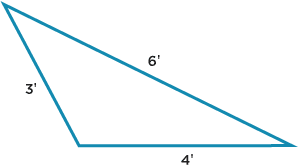# Polygons and Perimeters: Activity 1 of 3

## Directions

PERIMETER is the distance around a figure. To calculate the perimeter of a polygon, add up the lengths of its sides.

## Math18_A1_Q3.pngTo calculate the perimeter of a triangle (the total length of its 3 sides), use this formula: Perimeter = Side 1 + Side 2 + Side 3 , or P = S1+ S2 + S3.

Question 3 of 8